# McGraw Hill Math Grade 8 Lesson 8.2 Answer Key Subtracting Decimals

Practice the questions of McGraw Hill Math Grade 8 Answer Key PDF Lesson 8.2 Subtracting Decimals to secure good marks & knowledge in the exams.

Exercises

SUBTRACT

Question 1.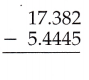11.9375
Explanation:
First line up all the decimals according to their place values,
then insert zeros in placeholders if needed to calculate.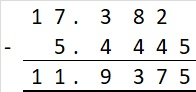Question 2.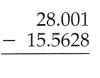12.4382
Explanation:
First line up all the decimals according to their place values,
then insert zeros in placeholders if needed to calculate.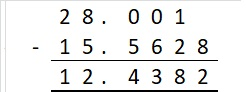Question 3.100.3899
Explanation:
First line up all the decimals according to their place values,
then insert zeros in placeholders if needed to calculate.Question 4.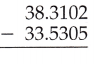4.7797
Explanation:
First line up all the decimals according to their place values,
then insert zeros in placeholders if needed to calculate.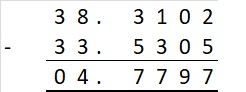Question 5.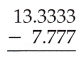5.5563
Explanation:
First line up all the decimals according to their place values,
then insert zeros in placeholders if needed to calculate.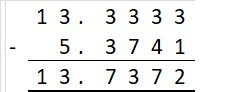Question 6.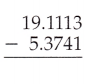13.7372
Explanation:
First line up all the decimals according to their place values,
then insert zeros in placeholders if needed to calculate.Question 7.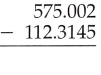462.6875
Explanation:
First line up all the decimals according to their place values,
then insert zeros in placeholders if needed to calculate.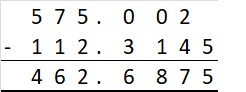Question 8.2.16069
Explanation:
First line up all the decimals according to their place values,
then insert zeros in placeholders if needed to calculate.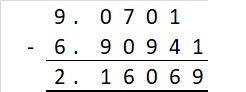Question 9.0.6683
Explanation:
First line up all the decimals according to their place values,
then insert zeros in placeholders if needed to calculate.Question 10.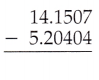8.94666
Explanation:
First line up all the decimals according to their place values,
then insert zeros in placeholders if needed to calculate.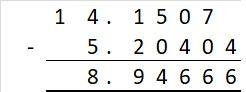Question 11.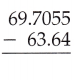6.0655
Explanation:
First line up all the decimals according to their place values,
then insert zeros in placeholders if needed to calculate.Question 12.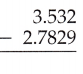0.7491
Explanation:
First line up all the decimals according to their place values,
then insert zeros in placeholders if needed to calculate.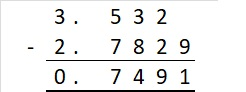Question 13.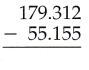124.157
Explanation:
First line up all the decimals according to their place values,
then insert zeros in placeholders if needed to calculate.Question 14.2.7392
Explanation:
First line up all the decimals according to their place values,
then insert zeros in placeholders if needed to calculate.Question 15.1.971
Explanation:
First line up all the decimals according to their place values,
then insert zeros in placeholders if needed to calculate.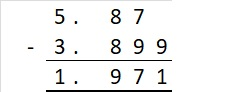Question 16.254.931
Explanation:
First line up all the decimals according to their place values,
then insert zeros in placeholders if needed to calculate.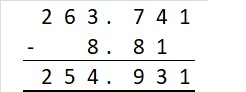Question 17.
The winner of the pole vault recorded a best vault of 5.8833 meters. The second-place winner recorded a best vault of 5.4993 meters. What was the difference between the winning vault and the second-place vault?
0.384 meters
Explanation:
The winner of the pole vault recorded a best vault of 5.8833 meters.
The second-place winner recorded a best vault of 5.4993 meters.
The difference between the winning vault and the second-place vault
5.8833 – 5.4993 = 0.384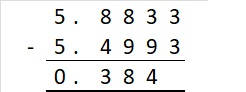Question 18.
Annie measured the depth of the water in the school’s fountain and found there were 9.774 inches of water. Annie measured the depth of the water again the next day and noted that the water level was 1.7456 inches lower. What was the new depth of the water?